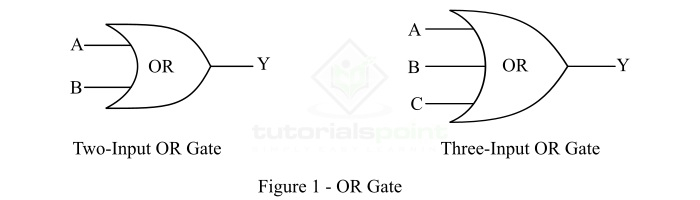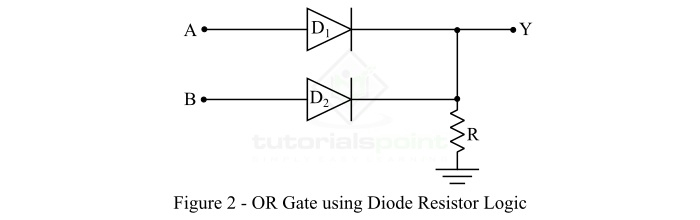# OR Gate Using Diode Resistor Logic

A logic gate is a digital electronic circuit that is used to implement different kinds of logical and comparison operations. We have several types of logic gates such as OR Gate, AND Gate, NOT Gate, NAND Gate, NOR Gate, etc. In this article, we will discuss the realization of OR Logic Gate using diode resistor logic, i.e. DL OR Gate. But before going into the realization of DL OR gate, let us discuss the basic of OR Gate.

## What is OR Gate?

OR Gate is a logic circuit which may have two or more binary inputs and gives an output according to the combination of the inputs.

The OR Gate produces a logic 1 (HIGH) output, even if one of its input is in the logic 1 (HIGH) state. The OR Gate gives a logic 0 (LOW) output, only when each one of its inputs is in the logic 0 (LOW) state. Therefore, the OR gate is also referred to as any or all gate. It may also be called as an inclusive OR gate because it includes the state where both the inputs can be present. The symbols of a two input and a three input OR gate are shown in Figure-1.## OR Operation

The OR operation is represented by ‘+’. The Boolean expression for the output of OR gate is given below,

$$\mathrm{Y = A+B+C+ \cdot \cdot \cdot }$$

Where, Y is the output of the OR Gate, A, B, C… are the input variables to the OR gate.

The operation of a logic gate is explained in terms of a truth table that represents the logical relationship between inputs and output. The following table is the truth table of a three input OR Gate.

Inputs Output
A B C Y = A + B + C
0 0 0 0
0 0 1 1
0 1 0 1
0 1 1 1
1 0 0 1
1 0 1 1
1 1 0 1
1 1 1 1

## OR Gate Using Diode Resistor Logic

We may realize the discrete OR gate by using diodes and resistors (called diode resistor logic). The circuit diagram of the OR Gate using diode resistor logic is shown in the following Figure-2.Here, the inputs A and B to the gates may be either 0 V or +5 V. The operation of the OR Gate using diode-resistor logic is explained as follows −

• When A = 0 V and B = 0 V, both the diodes D1 and D2 are off. Hence, there is no current flow through the resistor R, so there is no voltage drop across the resistor R. Consequently, the output voltage Y = 0 V.

• When A = +5 V and B = 0 V, then the diode D1 is on and the diode D2 is off. Thus, the output voltage Y = 5 V.

• When A = 0 V and B = +5 V, then the diode D1 is off and the diode D2 is on. Therefore, the output voltage Y = 5 V.

• When A = +5 V and B = +5 V, then both diodes D1 and D2 are on. Therefore, the output voltage Y = 5 V.

Note − In practice, there is a small voltage drop (about 0.7 V) takes place in diode itself. Hence, the output voltage Y = 5 – 0.7 = 4.3 V. This output voltage is regarded as logic 1.

## Conclusion

From the above discussion, we can conclude that the OR Gate is a basic logic gate that is used to implement the operation of binary sum. The OR gate gives a logic 1 as output even if any of its inputs is at logic 1 state. It gives logic 0 output, only when all its inputs are at logic 0 state.

Updated on: 26-Dec-2022

3K+ Views